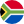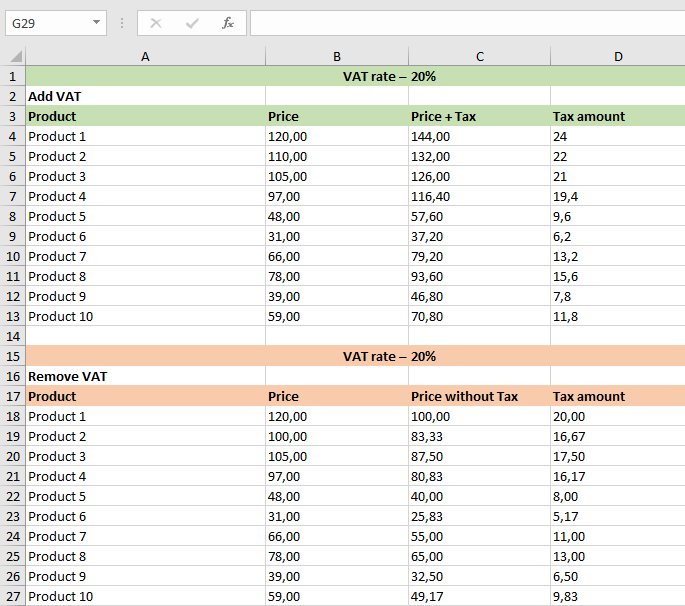# South African VAT Calculator

Add or remove VAT in South Africa in a click with our online VAT calculator. Just type the price and click the button.
This is some text inside of a div block.
Oops! Something went wrong while submitting the form.

How to use the VAT calculator

Calculating VAT for VAT-inclusive and VAT-exclusive prices has never been easier. Only 3 easy steps for VAT calculation:

• Check the VAT rate. It’s preset to 15%
• Enter the net or gross sum
• Click "Remove VAT" to deduct VAT from figure or "Add VAT" to calculate amount with VAT
VAT calculator preset to ZA rates
 🗺️ Country of VAT South Africa 💷 Currency of VAT Rand (R) 📈 Standard rate 15% VAT 📉 Reduced rate 0% VAT## What is VAT and how much is it in South Africa?

VAT (known in some countries as a GST) is a tax, which is payable on sales of goods or services within the territory of the Member States of the EU. The current standard rate of VAT is 15%. The reduced rates of VAT are 0%.

At sars.gov.za you can find information on how to register for, calculate, pay and reclaim VAT, VAT rates, and VAT on property rules by businesses that are VAT registered.

## How to work out VAT

Only 3 easy steps to calculate VAT onlineCheck the "VAT rate" (it’s pre-set to the SA VAT rate of 15%)Enter the amount – net or gross price. Check full VAT rates list belowClick "Remove VAT" or "Add VAT" depending on what you want

## How to calculate VAT in math or Excel

Following these simple steps can help you to calculate VAT backwards or forwards.If you want to add VAT to the price, you just need to divide the price by 100 and then multiply by (100 + VAT rate). That's all, you got the price including VAT - Gross price.### Removing VAT formula

If you want to subtract VAT from the price, you need to divide the price by (100 + VAT rate) and then multiply by 100.  Now you know the price excluding VAT - Net price.### VAT calculation in Excel

If you need to calculate VAT for several products at once, it is easier to use Excel. I created a template – download it, it's free.Antony Kidless